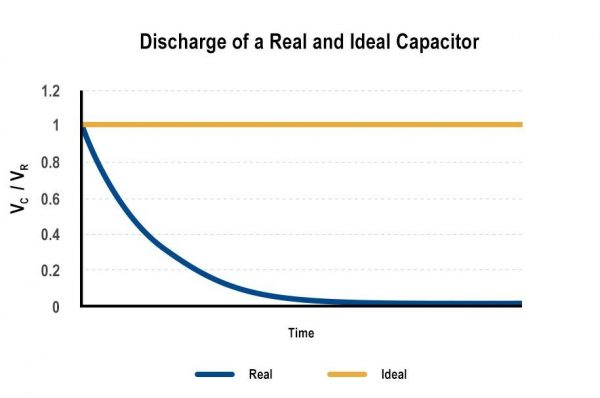# Shutting Down Safely with CapacitorsThe primary job of a capacitor is to store charge and later to release that charge for another part of the circuit. This charging and discharging behavior is determined by several factors – not just what is placed next to the capacitor, but also by some non-idealities within any real-world capacitor itself.

Ideal capacitors have relatively simple-to-understand energy storage characteristics. Unfortunately, those are complicated considerably by the real world. One common application of energy storage in capacitors is the safe shutdown of electronics when power is lost. Whether power is needed for a period of time long enough to safely shut down or to set the product into a safe condition, the requirements are almost the same.

## Ideal Capacitors

Ideal capacitors have no loss. They are purely reactive devices, without any resistive component to their impedance. Once fully charged, they hold their charge indefinitely. Unfortunately, ideal capacitors do not exist in the real world. But there is value in understanding the characteristics of ideal capacitors as a foundation for exploring how capacitors store energy.

Figure 1: A Basic Capacitor Charging Circuit

Capacitors store energy per the following equation:

Equation 1: Energy stored by an ideal capacitor

Where U = Energy (in Joules), Q = Charge (in Coulombs), C = Capacitance (in Farads), and V = Voltage (in Volts).

Once an ideal capacitor is fully charged by current from the supply, it will hold that charge indefinitely. If a resistive load is applied to an ideal capacitor holding a charge, the charge on the capacitor will provide current to the load and the voltage will discharge per the following equation.

Equation 2: Discharge of an ideal capacitor to a resistive load

## Real (non-ideal) Capacitors

As previously mentioned, however, ideal capacitors do not exist. The equivalent circuit of a real-life capacitor is more like this:

Real capacitors exhibit series resistance (ESR = Equivalent Series Resistance), parallel resistance (IR = Insulation Resistance), and series inductance (ESL = Equivalent Series Inductance), in addition to the ideal capacitance (C). These parasitic elements greatly complicate the energy storage and discharge behavior of capacitors.

The parallel insulation resistance (IR) is very large for most capacitors, on the order of several gigaohms (GΩ). But its very existence causes a non-ideal capacitor to self-discharge over time. Per Equation 2, the voltage on the capacitor will discharge to zero with IR as the load.

Figure 2: Discharge behavior of an ideal and real capacitor

Because of self-discharge, long-term energy storage in capacitors is not technologically realistic. Batteries, of course, experience a similar self-discharge of their stored energy due to internal resistance. But batteries can store thousands of times more energy chemically than capacitors of the same volume can in electric fields, making batteries preferred for longer term energy storage. Immediately upon power loss, however, there is still an opportunity for energy stored in capacitors to be discharged usefully, in a controlled fashion, to supply electronics for a short time.

## How DC Voltage Affects Charge Capacity

Different capacitor construction and material technologies cause capacitors to react differently to the application of a DC voltage. Multilayer ceramic (MLCC) class 2 capacitors, for example, use a ferroelectric dielectric which causes a reduction in capacitance as applied DC voltage increases. MLCC class 1 capacitors, however, use a paraelectric dielectric that remains stable over DC voltage. Polymer capacitors are similarly stable.

The practical effect of this is that some capacitors will experience a saturation of stored charge at a voltage lower than their maximum rated voltage. These capacitors are still able to operate up to their rated voltage, they just will not be storing the maximum possible charge – instead, the capacitance will drop and the charge will plateau.

Figure 3: Stored charge as DC voltage approaches maximum rated voltage for various capacitors

For power fail scenarios, this means selecting the proper capacitors is of critical importance. The designer has two options: select capacitors that are stable across DC voltage (i.e., using Cap 1 in the figure above) or understand the characteristics of the capacitor and derate the voltage accordingly (i.e., using Cap 2 in the figure above at <50% of its voltage rating).

Because of the effect of DC voltage on the capacitance of certain types of capacitors, the discharge of the example capacitors would vary depending on the amount of energy actually stored, rather than the labeled capacitance.

Figure 4: Discharge behavior is worse for capacitors storing less energy because of DC voltage

## Other Considerations: Temperature and ESR

Applied DC voltage is not the only factor in energy storage. Operating temperature and internal temperature also affect capacitance and lifetime.

Capacitors have temperature coefficients (TC), which indicate how much capacitance changes over temperature. Some capacitors have positive coefficients, meaning their capacitance increases as temperature increases, while most have negative coefficients, meaning their capacitance decreases as temperature increases.

Internal temperature, or self-heating, occurs primarily due to ESR and varies depending on the amount of ripple current or charge/discharge cycles in a given application.

## Conclusion

Capacitors can be an excellent solution for the energy storage needed to safely shut down electronics. Energy storage capacitors should be selected to have stable capacitance over the application’s desired temperature range, a derated voltage rating, and a very low ESR. Supercapacitors and electrolytics are often used for these types of applications.

For example application of energy storage calculations, is used the SSD Hold-Up Capacitor Calculator. SSDs require a certain amount of hold-up time upon power loss to flush the volatile cache and reach a safe shutdown state. This example uses T545 polymer electrolytic capacitors, which are designed for high energy applications.